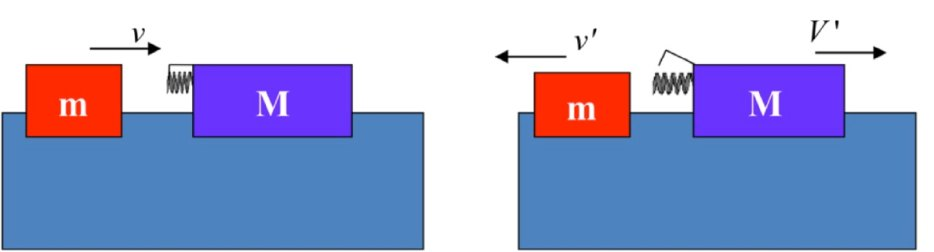Teaching Physics with the Physics Suite Edward F. Redish Problems Sorted by Type | Problems Sorted by Subject | Problems Sorted by Chapter in UP

A superelastic collision

Two gliders are set up to collide on an air track as shown in the figure below. The glider on the right is at rest and has a spring connected to it. Before the collision, as shown in the figure at the left, the smaller glider (mass m) is on the left approaching the larger glider (mass M). The spring on the larger glider is compressed and stores a spring potential energy of ΔE. When the two carts collide, the spring is released, giving all its potential energy up to the kinetic energy of the two carts. (The mass of the spring and latch is included in M; the friction internal to the spring and between the carts and the air track may be ignored.)a. Is the momentum of the two carts conserved in this collision? If it is, write the equation that the variables of this collision satisfy as a result using the symbols given in the description of the problem. If it is not, explain why not.

b. Is mechanical energy conserved in this collision? If it is, write the equation that the variables of this collision satisfy as a result using the symbols given in the description of the problem. If it is not, explain why not.